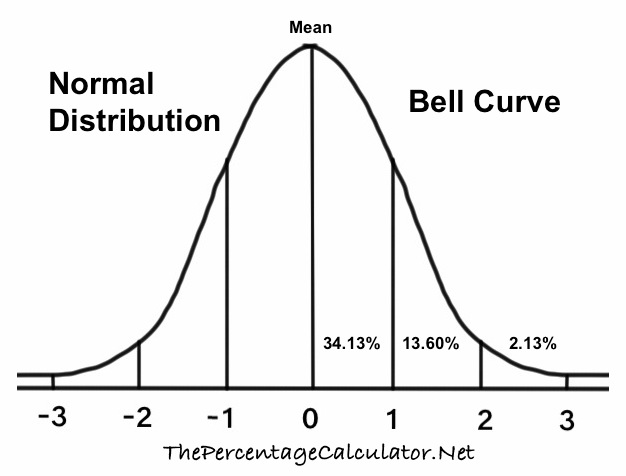Z Score 2.252We looked up the Z Score for 2.252 in our Normal Distribution Tables with Z Scores so you don't have to!

First, note that a Z Score of 2.252 means that your statistic is 2.252 standard deviation to the right of the mean on a bell curve. Here is a Bell Curve so you can visualize where 2.252 is on a bell curve.We used three different distribution tables, and we will give you the 2.252 Z Score probability, percentile, and explanations for all three.

Cumulative from mean
This gives you the probability of the area from the mean to your Z Score. The cumulative from-mean probability and percentile for a 2.252 Z score is displayed here:

0.487838863992018
= 48.7839%

Cumulative
This gives you the probability of the area below the Z Score. The cumulative probability and percentile for a 2.252 Z score is displayed here:

0.987838863992018
= 98.7839%

Complementary cumulative
This gives you the probability of the area above the Z Score. The complementary cumulative probability and percentile for a 2.252 Z Score is displayed here:

0.0121611360079817
= 1.2161%

Z Score Table Lookup
Here you can submit Z Scores between -3.999 and 3.999 for us to look up in our Normal Distribution Tables.

Z Score 2.253
Here is the next Z Score we looked up in our Normal Distribution Tables.

The percentiles on this page are rounded to the nearest 4 decimals.

Copyright  |   Privacy Policy  |   Disclaimer  |   Contact